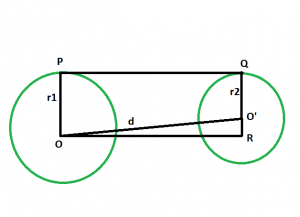# Length of direct common tangent between the two non-intersecting Circles

Given two circles, of given radii, have there centres a given distance apart, such that the circles don’t touch each other. The task is to find the length of the direct common tangent between the circles.

Examples:

```Input: r1 = 4, r2 = 6, d = 12
Output: 11.8322

Input: r1 = 5, r2 = 9, d = 25
Output: 24.6779
```

## Recommended: Please try your approach on {IDE} first, before moving on to the solution.Approach:

• Let the radii of the circles be r1 & r2 respectively.
• Let the distance between the centers be d units.
• Draw a line OR parallel to PQ
• angle OPQ = 90 deg
angle O’QP = 90 deg

{ line joining the centre of the circle to the point of contact makes an angle of 90 degrees with the tangent }
• angle OPQ + angle O’QP = 180 deg
OP || QR
• Since opposite sides are parallel and interior angles are 90, therefore OPQR is a rectangle.
• So OP = QR = r1 and PQ = OR = d
• In triangle OO’R

angle ORO’ = 90
By Pythagoras theorem

OR^2 + O’R^2 = (OO’^2)
OR^2 + (r1-r2)^2 = d^2

• so, OR^2= d^2-(r1-r2)^2
OR = √{d^2-(r1-r2)^2}• Below is the implementation of the above approach:

## C++

 `// C++ program to find ` `// the length of the direct ` `// common tangent between two circles ` `// which donot touch each other ` ` `  `#include ` `using` `namespace` `std; ` ` `  `// Function to find the length of the direct common tangent ` `void` `lengtang(``double` `r1, ``double` `r2, ``double` `d) ` `{ ` `    ``cout << ``"The length of the direct"` `         ``<<``" common tangent is "`  `         ``<< ``sqrt``(``pow``(d, 2) - ``pow``((r1 - r2), 2)) ` `         ``<< endl; ` `} ` ` `  `// Driver code ` `int` `main() ` `{ ` `    ``double` `r1 = 4, r2 = 6, d = 12; ` `    ``lengtang(r1, r2, d); ` `    ``return` `0; ` `} `

## Java

 `// Java program to find ` `// the length of the direct ` `// common tangent between two circles ` `// which donot touch each other ` `class` `GFG ` `{ ` ` `  `// Function to find the length of ` `// the direct common tangent ` `static` `void` `lengtang(``double` `r1, ``double` `r2, ``double` `d) ` `{ ` `    ``System.out.println(``"The length of the direct"` `        ``+``" common tangent is "` `        ``+(Math.sqrt(Math.pow(d, ``2``) -  ` `          ``Math.pow((r1 - r2), ``2``)))); ` `} ` ` `  `// Driver code ` `public` `static` `void` `main(String[] args)  ` `{ ` `    ``double` `r1 = ``4``, r2 = ``6``, d = ``12``; ` `    ``lengtang(r1, r2, d); ` `} ` `} ` ` `  `/* This code contributed by PrinciRaj1992 */`

## Python3

 `# Python3 program to find ` `# the length of the direct ` `# common tangent between two circles ` `# which do not touch each other ` `import` `math ` ` `  `# Function to find the length  ` `# of the direct common tangent ` `def` `lengtang(r1, r2, d): ` `    ``print``(``"The length of the direct common tangent is"``,  ` `         ``(((d ``*``*` `2``) ``-` `((r1 ``-` `r2) ``*``*` `2``)) ``*``*` `(``1` `/` `2``))); ` ` `  `# Driver code ` `r1 ``=` `4``; r2 ``=` `6``; d ``=` `12``; ` `lengtang(r1, r2, d); ` ` `  `# This code is contributed by 29AjayKumar `

## C#

 `// C# program to find  ` `// the length of the direct  ` `// common tangent between two circles  ` `// which donot touch each other  ` `using` `System; ` ` `  `class` `GFG  ` `{  ` ` `  `    ``// Function to find the length of  ` `    ``// the direct common tangent  ` `    ``static` `void` `lengtang(``double` `r1, ``double` `r2, ``double` `d)  ` `    ``{  ` `        ``Console.WriteLine(``"The length of the direct"` `            ``+``" common tangent is "` `            ``+(Math.Sqrt(Math.Pow(d, 2) -  ` `            ``Math.Pow((r1 - r2), 2))));  ` `    ``}  ` `     `  `    ``// Driver code  ` `    ``public` `static` `void` `Main()  ` `    ``{  ` `        ``double` `r1 = 4, r2 = 6, d = 12;  ` `        ``lengtang(r1, r2, d);  ` `    ``}  ` `}  ` ` `  `// This code is contributed by AnkitRai01 `

## PHP

 ` `

Output:

```The length of the direct common tangent is 11.8322
```

My Personal Notes arrow_drop_upCheck out this Author's contributed articles.

If you like GeeksforGeeks and would like to contribute, you can also write an article using contribute.geeksforgeeks.org or mail your article to contribute@geeksforgeeks.org. See your article appearing on the GeeksforGeeks main page and help other Geeks.

Please Improve this article if you find anything incorrect by clicking on the "Improve Article" button below.